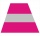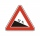# The mast

The top of the pole we see at an angle of 45°. If we approach the pole by 10 m, we see the top of the pole at an angle of 60°. What is the height of the pole?

Result

h =  23.66 m

#### Solution:

$\tan(60^\circ ) = h/x \ \\ \tan(45^\circ ) = h/(x+10) \ \\ \ \\ x = h / \tan(60^\circ ) = h \cdot \ t_{ 1 } \ \\ t_{ 1 } = 1/ \tan( (60^\circ \rightarrow rad) = 1/ \tan( (60 \cdot \ \dfrac{ \pi }{ 180 } \ ) = 0.57735026919 ) \ \\ t_{ 2 } = \tan( (45^\circ \rightarrow rad) = \tan( (45 \cdot \ \dfrac{ \pi }{ 180 } \ ) = 1 ) \ \\ \ \\ t_{ 2 } \cdot \ (h \cdot \ t_{ 1 }+10) = h \ \\ t_{ 2 } \cdot \ h \cdot \ t_{ 1 }+10 \cdot \ t_{ 2 } = h \ \\ \ \\ h = \dfrac{ 10 \cdot \ t_{ 2 } }{ 1-t_{ 2 } \cdot \ t_{ 1 } } = \dfrac{ 10 \cdot \ 1 }{ 1-1 \cdot \ 0.5774 } \doteq 23.6603 = 23.66 \ \text { m }$

Leave us a comment of this math problem and its solution (i.e. if it is still somewhat unclear...):Be the first to comment!## Next similar math problems:Mast has 13 m long shadow on a slope rising from the mast foot in the direction of the shadow angle at angle 15°. Determine the height of the mast, if the sun above the horizon is at angle 33°. Use the law of sines.
2. The hemisphereThe hemisphere container is filled with water. What is the radius of the container when 10 liters of water pour from it when tilted 30 degrees?
3. Hole's anglesI am trying to find an angle. The top of the hole is .625” and the bottom of the hole is .532”. The hole depth is .250” what is the angle of the hole (and what is the formula)?
4. RiverFrom the observatory 11 m high and 24 m from the river bank, river width appears in the visual angle φ = 13°. Calculate width of the river.
5. Water channelThe cross section of the water channel is a trapezoid. The width of the bottom is 19.7 m, the water surface width is 28.5 m, the side walls have a slope of 67°30' and 61°15'. Calculate how much water flows through the channel in 5 minutes if the water flow
6. ABCDAC= 40cm , angle DAB=38 , angle DCB=58 , angle DBC=90 , DB is perpendicular on AC , find BD and AD
7. Children playgroundThe playground has the shape of a trapezoid, the parallel sides have a length of 36 m and 21 m, the remaining two sides are 14 m long and 16 m long. Determine the size of the inner trapezoid angles.
8. A rhombusA rhombus has sides of length 10 cm, and the angle between two adjacent sides is 76 degrees. Find the length of the longer diagonal of the rhombus.
9. Isosceles triangle 10In an isosceles triangle, the equal sides are 2/3 of the length of the base. Determine the measure of the base angles.
10. The trapeziumThe trapezium is formed by cutting the top of the right-angled isosceles triangle. The base of the trapezium is 10 cm and the top is 5 cm. Find the area of trapezium.
11. Sides of right angled triangleOne leg is 1 m shorter than the hypotenuse, and the second leg is 2 m shorter than the hypotenuse. Find the lengths of all sides of the right-angled triangle.
12. A concrete pedestalA concrete pedestal has a shape of a right circular cone having a height of 2.5 feet. The diameter of the upper and lower bases are 3 feet and 5 feet, respectively. Determine the lateral surface area, total surface area, and the volume of the pedestal.
13. Secret treasureScouts have a tent in the shape of a regular quadrilateral pyramid with a side of the base 4 m and a height of 3 m. Determine the radius r (and height h) of the container so that they can hide the largest possible treasure.
14. Coordinates of square verticesI have coordinates of square vertices A / -3; 1/and B/1; 4 /. Find coordinates of vertices C and D, C 'and D'. Thanks Peter.
15. Cuboid face diagonalsThe lengths of the cuboid edges are in the ratio 1: 2: 3. Will the lengths of its diagonals be the same ratio? The cuboid has dimensions of 5 cm, 10 cm, and 15 cm. Calculate the size of the wall diagonals of this cuboid.
16. Body diagonalCalculate the volume of a cuboid whose body diagonal u is equal to 6.1 cm. Rectangular base has dimensions of 3.2 cm and 2.4 cm
17. Uphill gardenI have a garden uphill, increasing from 0 to 4.5 m for a length of 25 m, how much is the climb in percent?